Next: Worked example 6.3: Skater Up: Conservation of momentum Previous: Worked example 6.1: Cannon

## Worked example 6.2: Hitting a softball

Question: A softball of mass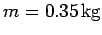is pitched at a speed of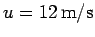. The batter hits the ball directly back to the pitcher at a speed of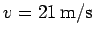. The bat acts on the ball for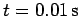. What impulse is imparted by the bat to the ball? What average force is exerted by the bat on the ball?

Answer: The initial momentum of the softball is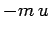, whereas its final momentum is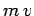. Here, the final direction of motion of the softball is taken to be positive. Thus, the net change in momentum of the softball due to its collision with the bat is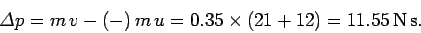By definition, the net momentum change is equal to the impulse imparted by the bat, so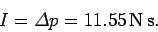The average force exerted by the bat on the ball is simply the net impulse divided by the time interval over which the ball is in contact with the bat. Hence,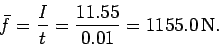Next: Worked example 6.3: Skater Up: Conservation of momentum Previous: Worked example 6.1: Cannon
Richard Fitzpatrick 2006-02-02